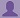SHARE
HELP

To start with, Sr2Jr’s first step is to reduce the expenses related to education. To achieve this goal Sr2Jr organized the textbook’s question and answers. Sr2Jr is community based and need your support to fill the question and answers. The question and answers posted will be available free of cost to all.Authors:
J. David Irwin, R. Mark Nelms
Chapter:
Basic Concepts
Exercise:
Problems
Question:1 | ISBN:9780470633229 | Edition: 10Question

If the current in an electric conductor is 2.4 A, how many coulombs of charge pass any point in a 30-second interval?Answer

Given data:

current I = 2.4 sec

Time t= 30 sec

Charge Q= ?

We know that current = charge / time

I = Q/t

Q = I * t

Q =  30 sec*2.4 A

=   72C

The amount of charge that passes any point in a 30 sec time is 72C.

Discussions

Post the discussion to improve the above solution.